InequalitiesOverview

Expect to see some questions on the topic of Inequalities on the SAT. In this section, we will review the following aspects of this topic:

• Definition of an Inequality
• Solving Inequalities
• Graphing Inequalities

Let’s look at each of these in more detail:

Definition of an Inequality

Inequalities are used to compare and order quantities. When comparing two quantities, use inequality symbols to show the relationship between the quantities.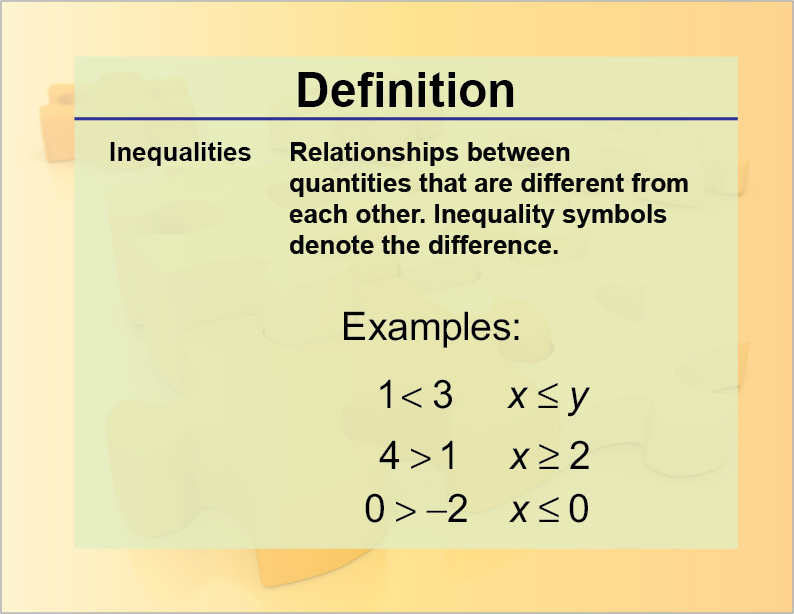Inequalities on a Number Line

You know that 2 < 5. This is how this inequality is represented on a number line.In fact, this number line represents two inequalities:Now take a look at this number line.This number line represents these two inequalities:Notice that numbers are arranged on a number line from least to greatest in going from left to right. Take a look at this number line:This number line results in these inequalities:Inequalities with Variables

So far you’ve seen examples of inequalities that represent numerical values. Inequalities can also be used to represent an unknown value x.

Take a look at this inequality:What is says is that x is any value greater than, but not including 1. Representing this inequality on a number line is to highlight all numbers to the right of 1.The solid red line to the right of 1 means that x can equal any of the values on this red line. The open circle on 1 means that x cannot equal 1. The arrow head indicates that there are an infinite number of possible values of x.

If we change the inequality to the one shown below, then the graph changes.A “greater than or equal” inequality includes a solid circle at x = 1.

Here is a “less than or equal to” inequality:Now look at this inequality:This inequality says that x can be any value greater than (but not equal to) 1 and less than (but not equal to) 6. This is what it looks like on a number line:This is known as a compound inequality.

Here is the version of the inequality that includes the closed-circle endpoints:So far, the examples we’ve looked at have a single variable. There are also inequalities that have two variables, like the one shown below:Here is the graph of the inequality: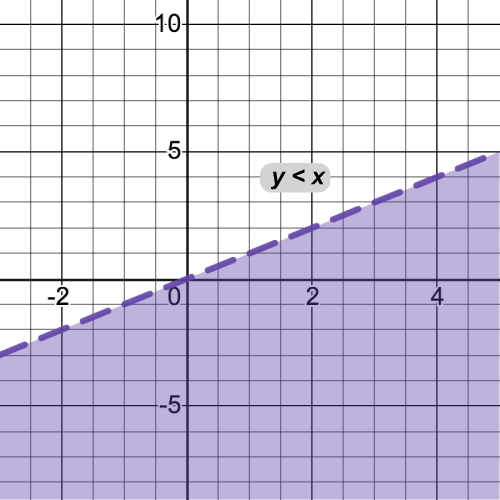The dashed line is the equivalent of the open circle on the single variable graph. The shading below the line represents the allowed values for x and y.

Here is the graph of the “less than or equal to” version.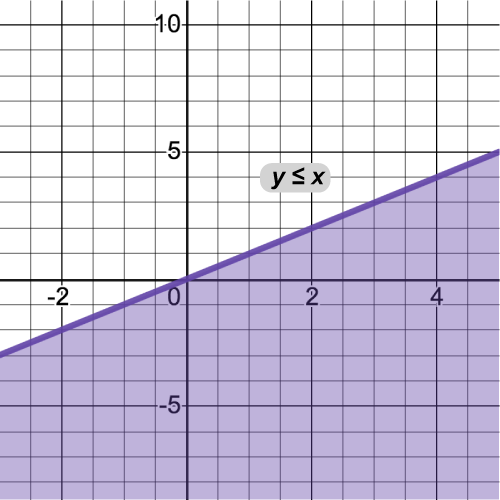Note the solid line for this graph.

Here are the “greater than” graphs.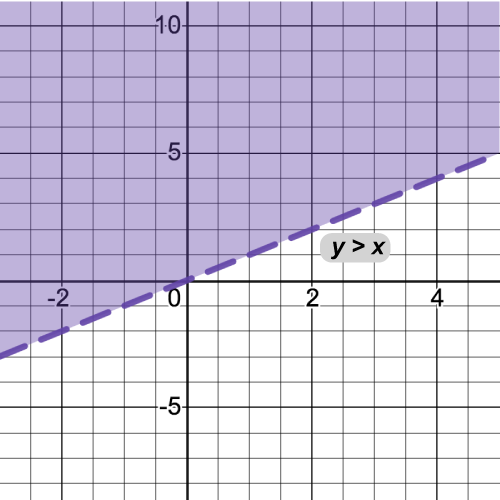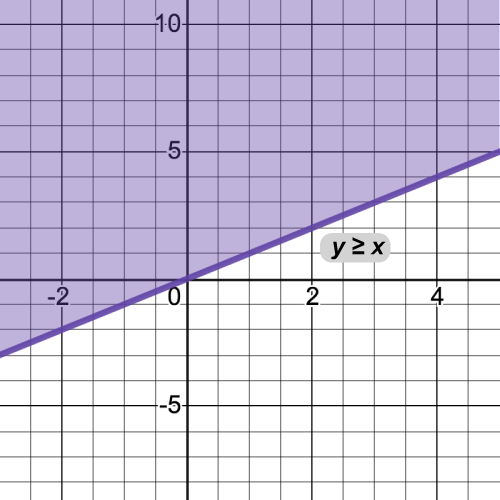Solving Inequalities: One Variable

Solving an inequality is similar to solving an equation. The results, however, have different meanings.

Look at this equation and its solution.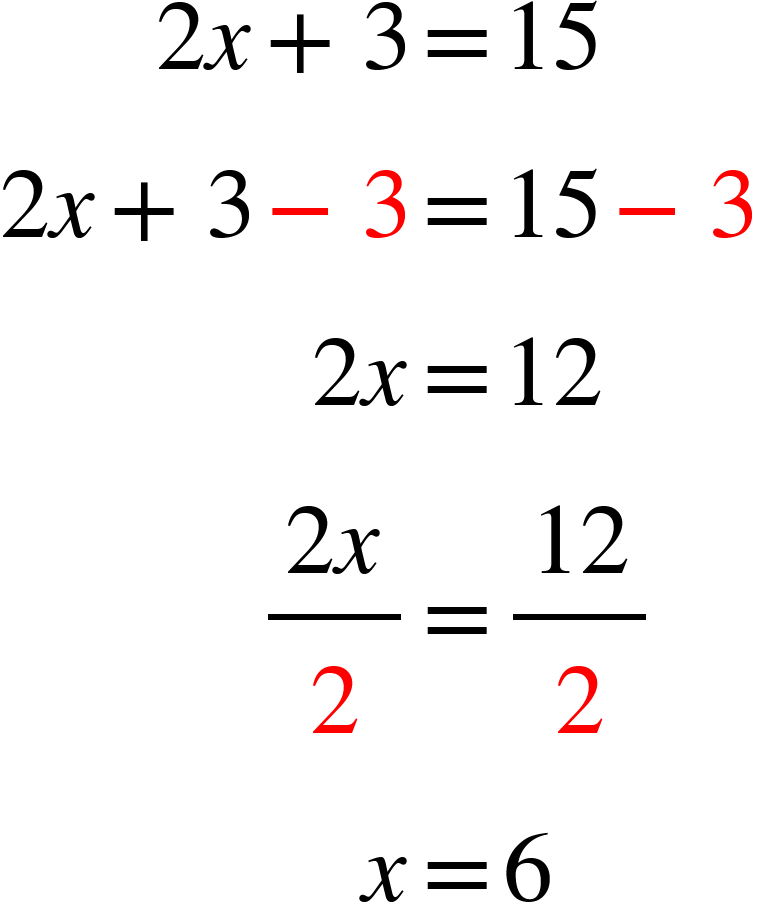Now look at the same solution in the form of an inequality: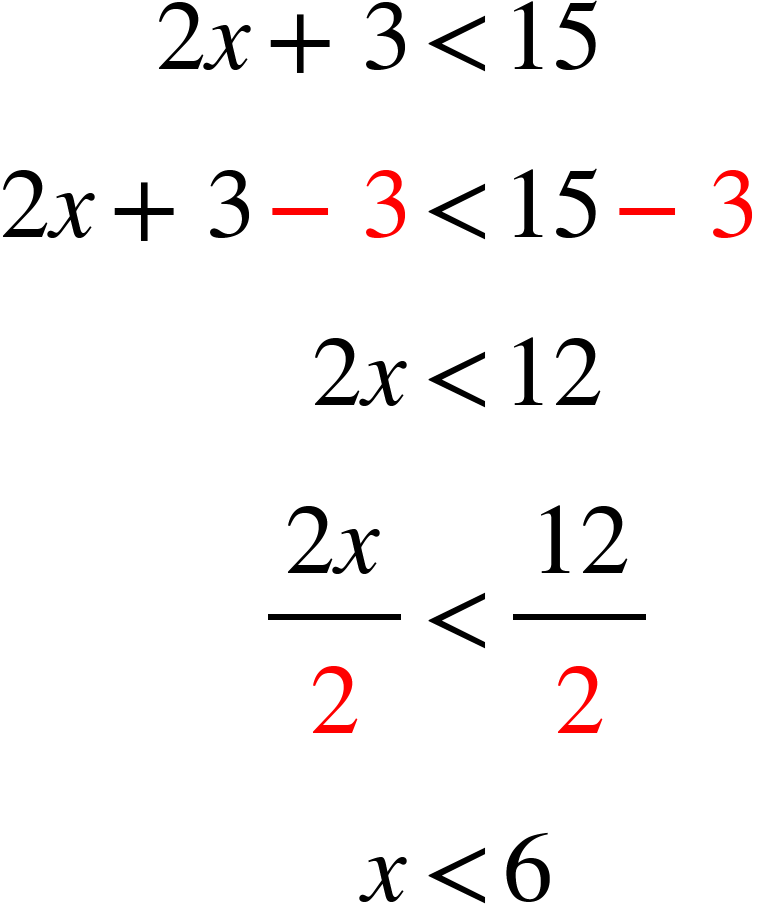Let’s compare the solution to the equation and the solution to the inequality:

 Equation:Inequality:Single solution Infinite number of solutionsNow look at this version of the equation and inequality: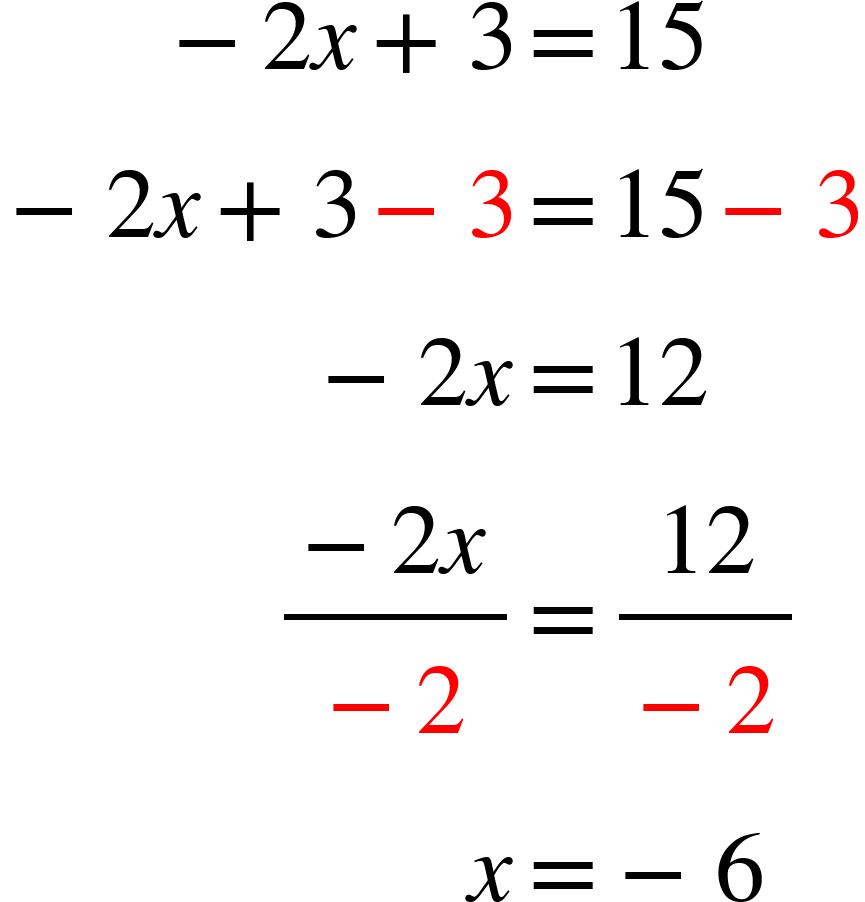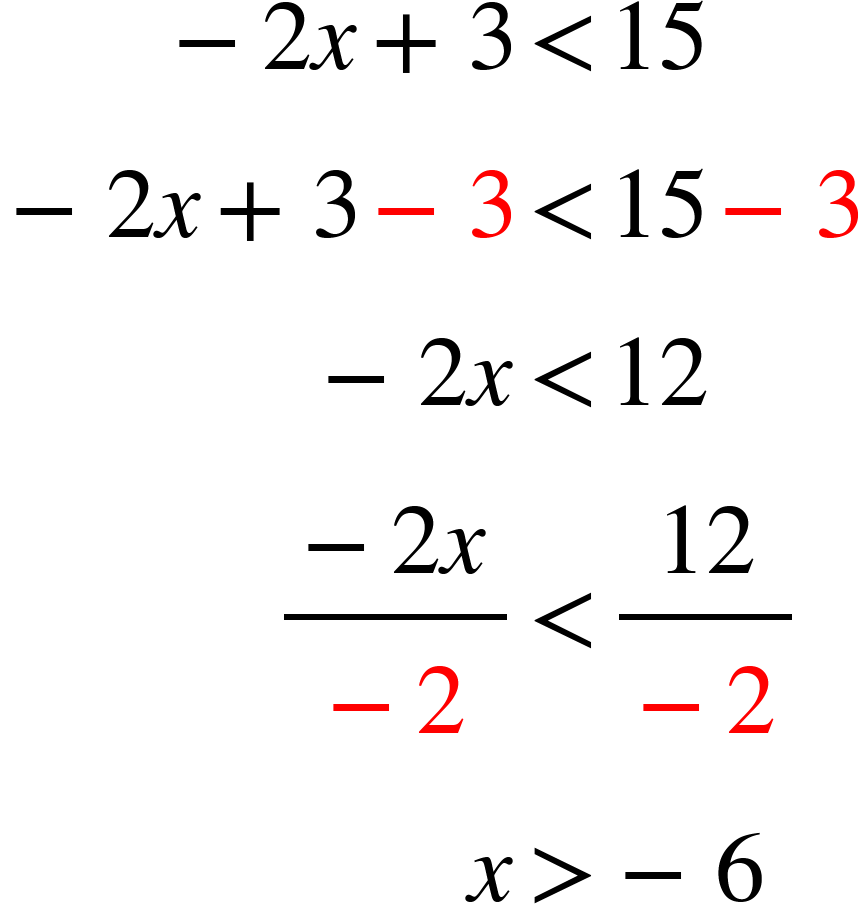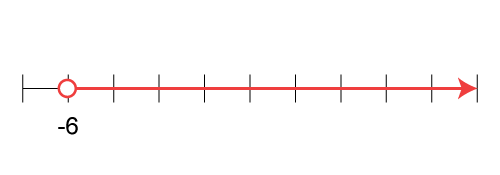Notice that the inequality changed from a “less than” to a “greater than”. The inequality sign changes when multiplying or dividing by a negative number.

To see examples of solving inequalities, click on this link.

 SAT Skill: Solving Inequalities Example 1 Is 1 a possible solution to this inequality?Solve the inequality and graph it: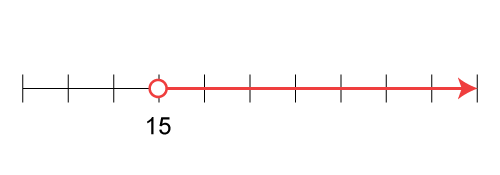Since 1 is to the left of 15 on the number line, it cannot be a solution to the inequality. Example 2 Is 5 a possible solution to this inequality?Solve the inequality and graph it:Since -5 is to the left of -2, then it is a possible solution.

Solving Inequalities: Two Variables

Solving equations can also involve two variables. Here is an example:Solve this inequality as if it were an equation:Notice that the inequality is written in slope-intercept form. Here is the graph of it.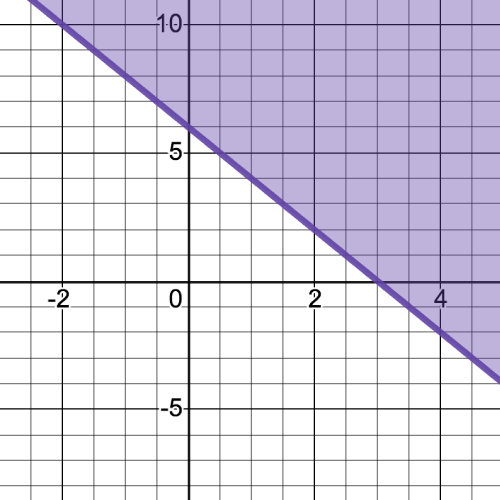Expect to see an SAT question that involves a system of inequalities. Here is an example.

Find a possible solution to this system of inequalities:Graph the inequalities and find where the graphs of the inequalities overlap.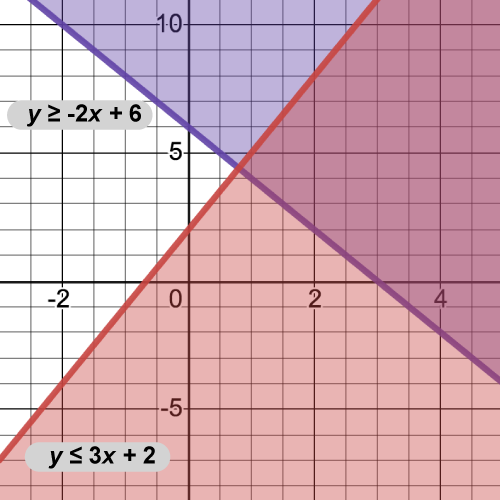The dark shaded region is the region of overlap. Any coordinates in this region are possible solutions to the inequality. For example the coordinates (4, 5) are a solution to the system of inequalities.

You don’t need a graphing calculator to graph these types of inequalities. Here’s a simple method for graphing an inequality. Let’s look at this inequality:Think of the graph of the linear equation y = 2x + 4. The y-intercept is 4, so graph that.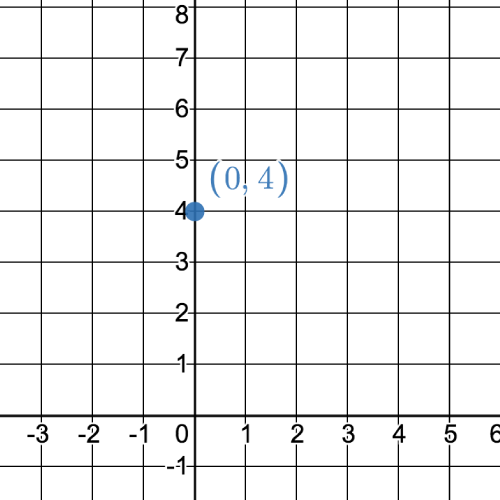We know the line has a slope of 2, so starting at the y-intercept, count 2 spaces up and 1 to the right. Draw a line connecting those points.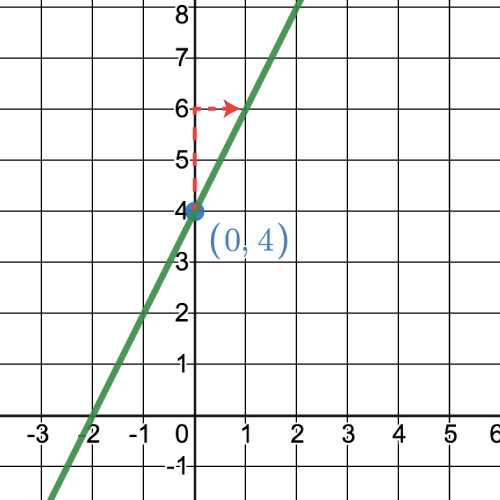Finally, since the inequality is “>”, then make the line a dashed line and shade everything above that line.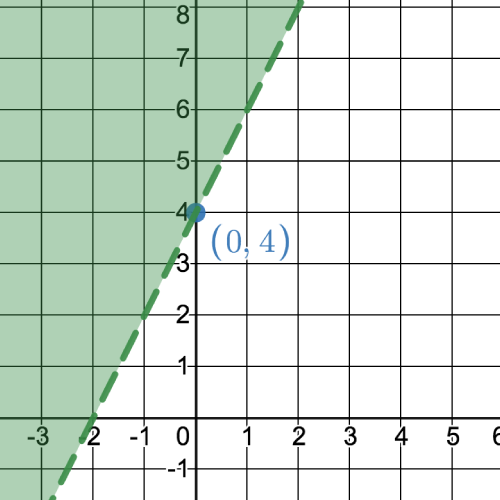SAT Skill: Solving Inequalities in Two Variables Example 1 Is the origin a possible solution to this system of inequalities?Sketch the graph of the inequalities and find the region of overlap.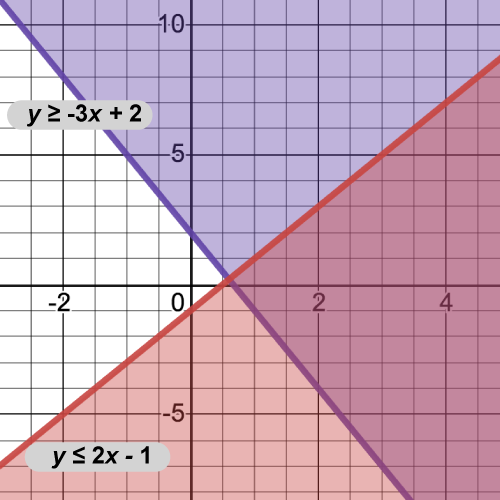Since the origin is not in the overlap region, then it cannot be a possible solution to the system of inequalities.